Editor Ratings:
User Ratings:
[Total: 0 Average: 0]

Tibi’s Mathematics Suite is a free application which allows you to perform some of the major mathematical functions and thus eliminate the need of any manual calculations. This suite works one step ahead of the traditional calculators by offering you a variety of functions such as factorization, matrix calculation, graphing calculator and scientific calculator. Of course, this is is a good deal for our students and others who wish to perform some basic functions to avoid any manual calculations. Let’s check out how this application might help you!

## Using Tibi’s Mathematics Suite

To begin using this application, you need to download and install the application. Once the application starts running you can see it in the task bar. Simply, right click the application to choose any function.As you can see, choosing any function, launches the application window for that particular application.

Also check out free math learning websites reviewed by us.

## Factorizator

Using Factorizator is simple. All you need to do is enter the number in the space provided. On factorization, as you would do in the conventional method, the remainder and divisors are computed so that you can list the factors. However, the time taken to compute also depends on the number of digits.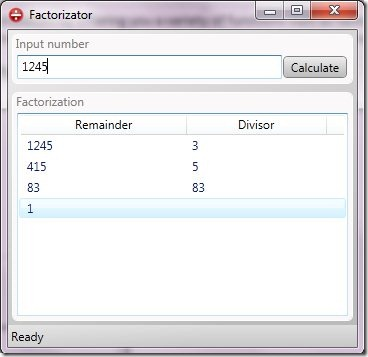## Graphing Calculator

One of the most interesting features of Tibi’s Mathematics Suite is the graphing calculator. As the name suggests, this functions closely with graphs and you can plot them, evaluate them or even integrate them. Importing expressions is also not a big deal! So you understand how powerful this function can be. We took a basic function ‘2x+5’ and plotted a graph using this suite. This can be a great help for the kids who have a tough time plotting graphs in their maths homework!Now, integrate the expression. However the software warns that the results might not be accurate. You can set the integration limits too.## Matrix calculator

Once again, another innovative feature present in the suite, Matrix calculator would be a respite to most of the students who dread completing their Matrix questions. Let’s have a look at what Matrix calculator can do for you.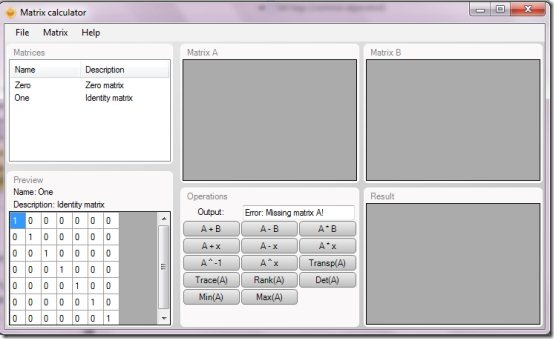As you can see in the snapshot above, we can consider two matrices at a time. The preview for identity matrix and zero matrix can be seen on the left and can accrodingly be adjusted to add/delete rows and columns. Once you add matrices to slot A and B, you can perform any operation as listed in the window above. For performing operations on a single matrix, you just need to add the matrix to a single slot.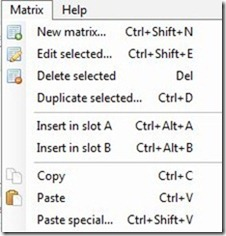The above snapshot captures the Matrix menu which can help you perform loads of tasks. Explore and you can perform almost anything with the matrix calculator. Suppose, we wish to add two matrices. Now we chose the option ‘New Matrix’ from the menu to create our own data. We created a 3×3 matrix. We clicked on ‘apply’ and the matrix was added to the matrices present on left panel.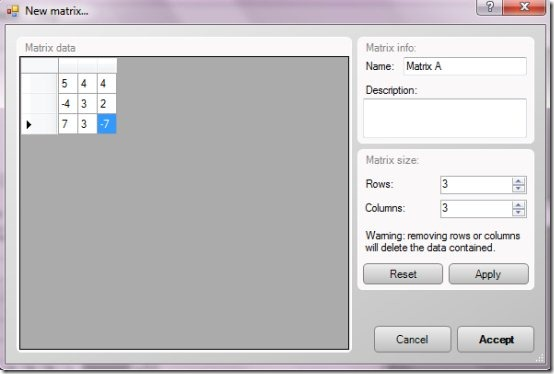Similarly, we created another 3×3 matrix and added to our matrix list. The next task was to insert the matrices in their respective slots.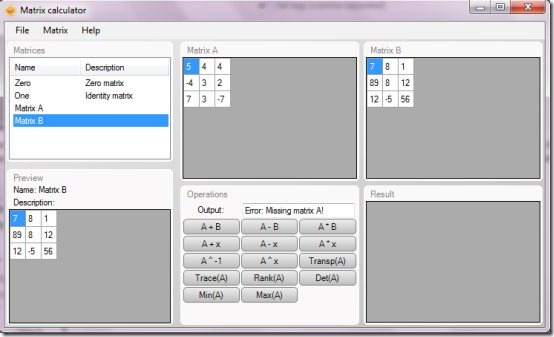Now, we will click on the required operation to display the result, in this case being ‘A*B’.## Scientific calculator

Finally, this suite offers you the scientific calculator to make your calculations far easier with a number of functions as compared to the traditional calculator.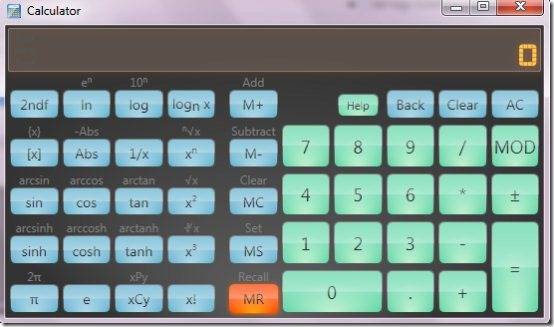## Verdict

As you might have understood by now, this suite makes lots of functions easier. So you can definitely give it a try!